GATE  >  Agricultural Engineering- AG 2013 GATE Paper (Practice Test)

# Agricultural Engineering- AG 2013 GATE Paper (Practice Test) - GATE

Test Description

## 65 Questions MCQ Test GATE Past Year Papers for Practice (All Branches) - Agricultural Engineering- AG 2013 GATE Paper (Practice Test)

Agricultural Engineering- AG 2013 GATE Paper (Practice Test) for GATE 2023 is part of GATE Past Year Papers for Practice (All Branches) preparation. The Agricultural Engineering- AG 2013 GATE Paper (Practice Test) questions and answers have been prepared according to the GATE exam syllabus.The Agricultural Engineering- AG 2013 GATE Paper (Practice Test) MCQs are made for GATE 2023 Exam. Find important definitions, questions, notes, meanings, examples, exercises, MCQs and online tests for Agricultural Engineering- AG 2013 GATE Paper (Practice Test) below.
Solutions of Agricultural Engineering- AG 2013 GATE Paper (Practice Test) questions in English are available as part of our GATE Past Year Papers for Practice (All Branches) for GATE & Agricultural Engineering- AG 2013 GATE Paper (Practice Test) solutions in Hindi for GATE Past Year Papers for Practice (All Branches) course. Download more important topics, notes, lectures and mock test series for GATE Exam by signing up for free. Attempt Agricultural Engineering- AG 2013 GATE Paper (Practice Test) | 65 questions in 180 minutes | Mock test for GATE preparation | Free important questions MCQ to study GATE Past Year Papers for Practice (All Branches) for GATE Exam | Download free PDF with solutions
 1 Crore+ students have signed up on EduRev. Have you?
Agricultural Engineering- AG 2013 GATE Paper (Practice Test) - Question 1

### Q. 1 – Q. 5 carry one mark each If 3 ≤ X ≤ 5 and  8 ≤  X  ≤ 11 then which of the following options is TRUE?

Agricultural Engineering- AG 2013 GATE Paper (Practice Test) - Question 2

### The Headmaster ___________ to speak to you. Which of the following options is incorrect to complete the above sentence?

Agricultural Engineering- AG 2013 GATE Paper (Practice Test) - Question 3

### Mahatama Gandhi was known for his humility as

Agricultural Engineering- AG 2013 GATE Paper (Practice Test) - Question 4

"All engineering students" "should learn mechanics", "mathematics and" "how to do computation."
I                                          II                                 III                                 IV
Which of the above underlined parts of the sentence is not appropriate?

Agricultural Engineering- AG 2013 GATE Paper (Practice Test) - Question 5

Select the pair that best expresses a relationship similar to that expressed in the pair: water: pipe::

Agricultural Engineering- AG 2013 GATE Paper (Practice Test) - Question 6

Q. 6 to Q. 10 carry two marks each.

Velocity of an object fired directly in upward direction is given by  = 80 −32    , where   (time) is in seconds. When will the velocity be between 32 m/sec  and 64 m/sec?

Agricultural Engineering- AG 2013 GATE Paper (Practice Test) - Question 7

In a factory, two machines M1 and M2 manufacture 60% and 40% of the autocomponents respectively. Out of the total production, 2% of M1 and 3% of M2 are found to be defective. If a randomly drawn autocomponent from the combined lot is found defective, what is the probability that it was manufactured by M2?

Agricultural Engineering- AG 2013 GATE Paper (Practice Test) - Question 8

Following table gives data on tourists from different countries visiting India in the year 2011.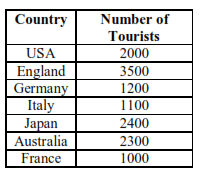Which two countries contributed to the one third of the total number of tourists who visited India in 2011 ?

Agricultural Engineering- AG 2013 GATE Paper (Practice Test) - Question 9

If |−2X + 9| = 3 then the possible value of |−X | −X2 would be:

Agricultural Engineering- AG 2013 GATE Paper (Practice Test) - Question 10

All professors are researchers , Some scientists are professors

Which of the given conclusions is logically valid and is inferred from the above arguments:

Agricultural Engineering- AG 2013 GATE Paper (Practice Test) - Question 11

Q. 11 – Q. 35 carry one mark each.

If P = AxB , where A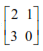and B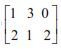then is

Agricultural Engineering- AG 2013 GATE Paper (Practice Test) - Question 12

If C= A×B , where A= 2i - j +3k  and B= i +2j ; then C is

Agricultural Engineering- AG 2013 GATE Paper (Practice Test) - Question 13

The general solution of the differential equation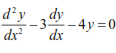is

Agricultural Engineering- AG 2013 GATE Paper (Practice Test) - Question 14

Eigenvalues of the matrix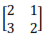is

Agricultural Engineering- AG 2013 GATE Paper (Practice Test) - Question 15

Poisson distribution having a mean of 5 will have √5 as

Agricultural Engineering- AG 2013 GATE Paper (Practice Test) - Question 16

During the testing of a spike-tooth type thresher for wheat crop, the throughput and the thresher capacity were found to be 750 kg h-1 and 445 kg h-1 , respectively. The grain-straw ratio (grain :straw) of the crop is 1.5 : 1. Material other than grain (MOG) collected at the main grain outlet is 0.5%. The total grain loss in percentage from all the sources will be

Agricultural Engineering- AG 2013 GATE Paper (Practice Test) - Question 17

The rear furrow wheel in a tractor mounted disc plough is provided to

Agricultural Engineering- AG 2013 GATE Paper (Practice Test) - Question 18

The cumulative discharge of a tractor mounted hydraulic sprayer having  7 nozzles is 2.0 L min-1 when the tractor is operated at 4 km h-1. If the nozzle spacing is 0.3 m, the discharge in L ha-1 is

Agricultural Engineering- AG 2013 GATE Paper (Practice Test) - Question 19

A two-wheel drive tractor, while negotiating a terrain, indicates 100% slip of one of the rear wheels. Under such a condition, the use of differential lock causes

Agricultural Engineering- AG 2013 GATE Paper (Practice Test) - Question 20

A double acting hydraulic cylinder has a rod diameter equal to one-half the piston diameter. If the system pressure is maintained constant, the ratio of load carrying capacity of extension stroke to that of retraction stroke is

Agricultural Engineering- AG 2013 GATE Paper (Practice Test) - Question 21

During a test, sound level was measured as 90 dB in the operator’s cabin on a tractor. Taking reference sound pressure as 2 x10-5 N m-2, the measured RMS sound pressure in N m is

Agricultural Engineering- AG 2013 GATE Paper (Practice Test) - Question 22

During land leveling of agricultural land for irrigation and drainage purposes, the acceptable deviation in elevation from the design value in metre is

Agricultural Engineering- AG 2013 GATE Paper (Practice Test) - Question 23

The gridiron pipe drainage system is more economical than the herringbone pipe drainage system because

Agricultural Engineering- AG 2013 GATE Paper (Practice Test) - Question 24

If the drainable porosity of a command area is 5% and the design rate of drop of the water table is 0.25 m day-1, the drainage coefficient of the command area in mm day-1 will be

Agricultural Engineering- AG 2013 GATE Paper (Practice Test) - Question 25

A soil has a void ratio of 0.75 and a specific gravity of 2.66. The value of critical hydraulic gradient at which quick sand condition will occur is

Agricultural Engineering- AG 2013 GATE Paper (Practice Test) - Question 26

The pressure that does not have any measurable influence on the void ratio or shearing resistance of the soil mass is

Agricultural Engineering- AG 2013 GATE Paper (Practice Test) - Question 27

The Rational method is used to estimate

Agricultural Engineering- AG 2013 GATE Paper (Practice Test) - Question 28

Pan evaporation data recorded at a certain location over a period of one week are 4.0, 4.3, 4.6, 4.9, 5.12, 5.18, and 6.21 mm. If irrigation scheduling based on ratio of irrigation water (IW) to cumulative pan evaporation (CPE) is practiced, the depth of irrigation at an interval of a week for IW/CPE = 0.9 is

Agricultural Engineering- AG 2013 GATE Paper (Practice Test) - Question 29

A 16 m high wind break is constructed to protect soil from wind erosion due to wind velocity of 18 m s-1 at 15 m height. The minimum wind velocity at 15 m height capable of moving the soil fraction is 8 m s-1. The angle of deviation of prevailing wind direction from the perpendicular to the wind break is 30º. The distance of full protection from the wind break in m is

Agricultural Engineering- AG 2013 GATE Paper (Practice Test) - Question 30

Milk enters into the heating section of a high temperature short time (HTST) pasteurization plant at a temperature of 45ºC and leaves at 72ºC. Hot water at temperature of 95 ºC enters countercurrently into the heat exchanger and leaves at 77ºC. The effectiveness of the heat exchanger is

Agricultural Engineering- AG 2013 GATE Paper (Practice Test) - Question 31

The equation representing the heat of respiration (q) of fruits and vegetables as a function oftemperature (θ) with positive constants a and b is

Agricultural Engineering- AG 2013 GATE Paper (Practice Test) - Question 32

For an initial spore load equal to 25 spores per container inoculated with Clostridium botulinum having D121 = 0.25 min, the spoilage probability of the container subjected to F121 = 1.5 min is

Agricultural Engineering- AG 2013 GATE Paper (Practice Test) - Question 33

Slope and intercept of the BET equation relating [aw / x(l - aw)] and aw is water activity
and x is the moisture content on dry basis, are 18 and 2, respectively. The values of thermodynamic constant C and BET monolayer moisture content C and BET monolayer moisture content xm (%) are respectively

Agricultural Engineering- AG 2013 GATE Paper (Practice Test) - Question 34

A cold storage chamber is constructed with 10 mm mortar, 200 mm brick, 100 mm insulation and 5 mm wood-board having thermal conductivities of 0.8, 1.5, 0.025 and 0.2 W m-1 K-1 , respectively. The resistance of 4 K mW-1 is offered by

Agricultural Engineering- AG 2013 GATE Paper (Practice Test) - Question 35

The horse power of the motor running the compressor of a refrigerator having COP of 4.5 and extracting 200 kJ kg-1 of evaporating heat with 1.5 kg min-1 refrigerant flow rate is

*Answer can only contain numeric values
Agricultural Engineering- AG 2013 GATE Paper (Practice Test) - Question 36

Q. 36 to Q. 65 carry two marks each.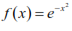for x = 1.1, 1.2, 1.3, 1.4 and 1.5, the value of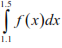by Simpson’s 1/3rd rule is __________

*Answer can only contain numeric values
Agricultural Engineering- AG 2013 GATE Paper (Practice Test) - Question 37

A tractor operated single acting trailing type disc harrow has 8 discs on each gang. The gang angle of both the gangs is maintained at 35º. The horizontal component of resultant soil reaction force on each disc is 600 N and it makes an angle of 30º with the gang axis. If  the  speed of operation is 6 km h-1, the required drawbar power in kW to operate the harrow will be _______________

*Answer can only contain numeric values
Agricultural Engineering- AG 2013 GATE Paper (Practice Test) - Question 38

A two-wheel drive tractor pulls an implement that has a draft force of 11.5 kN. The total motion resistance of the tractor is 2.5 kN. Under these circumstances, the slip of the drive wheels is 20%. If the power loss in transmission is 20%, the percentage of power lost in converting engine power into drawbar power is _______________

*Answer can only contain numeric values
Agricultural Engineering- AG 2013 GATE Paper (Practice Test) - Question 39

An unconfined aquifer extends over an area of 1 kmand has hydraulic conductivity, total porosity and specific retention of 20 m per day, 30% and 10%, respectively. After pumping some groundwater from this aquifer, the water table dropped to a depth of 20m from the ground level. If the water table was initially at 14.5 m below the ground level, the change in groundwater storage in million cubic meters would be _______________

*Answer can only contain numeric values
Agricultural Engineering- AG 2013 GATE Paper (Practice Test) - Question 40

Two parallel canals 50 m apart fully penetrate a homogeneous unconfined aquifer resting on a horizontal impermeable layer. The aquifer has a hydraulic conductivity of 3m day-1 and an effective porosity of 0.25. One-dimensional steady groundwater flow occurs from the upper canal to the lower canal with the height of water levels in the canals 10 m and 8.5 m from the aquifer bottom, respectively. If a sediment layer of 4 cm thick with hydraulic conductivity of 1.2 × 10-2 m day-1 is ultimately deposited on the inflow face, the groundwater discharge per 1000m width between the two canals in m3 day-1 will be _______________

*Answer can only contain numeric values
Agricultural Engineering- AG 2013 GATE Paper (Practice Test) - Question 41

The overall heat transfer coefficient based on the outside surface area of a tubular heat exchanger decreased due to fouling during operation from 1000 W m-2 K-1 to 800 W m-2 K-1. The fouling film coefficient of the heat exchanger in W m-2 K-1 is ________

*Answer can only contain numeric values
Agricultural Engineering- AG 2013 GATE Paper (Practice Test) - Question 42

A high pressure dairy homogenizer operates under upstream and downstream pressures of 200 and 40 bar respectively homogenizing 30 L of whole milk per hour. Density and specific heat capacity of whole milk are 1030 kg m–3 and 3.8 kJ kg–1 K–1, respectively.  Assuming complete energy conservation, the temperature rise of whole milk in degree Celsius is ____________

*Answer can only contain numeric values
Agricultural Engineering- AG 2013 GATE Paper (Practice Test) - Question 43

A fish fillet of 5 mm thickness having 85% moisture (wet basis) is to be frozen using a plate freezer. The plates are at ?35 ºC and the heat transfer coefficient between the fillet and the freezer plates can be assumed to be 2.0 W m-2 K-1 . The initial freezing temperature of fish is -2.5 ºC, latent heat of fusion is 330 kJ kg–1 ,density of fish is 1100 kg m–3 and thermal conductivity of frozen fish is 1.5 W m-1 K-1. The time required to freeze the fillet from the initial freezing temperature in hour(s) is _____

Agricultural Engineering- AG 2013 GATE Paper (Practice Test) - Question 44

Box 1 contains 15 balls out of which 3 are red.  Box 2 contains 12 balls out of which 4 are red.  If one ball is drawn at random from each box simultaneously, the probability of getting at least one red ball is

Agricultural Engineering- AG 2013 GATE Paper (Practice Test) - Question 45

A hemispherical vessel of 300 mm diameter is completely filled with oil and water. If the oil layer is 50 mm deep on the top, the volume of water in the vessel in litres is

Agricultural Engineering- AG 2013 GATE Paper (Practice Test) - Question 46

A tractor mounted off-set type reciprocating mower is driven by the PTO shaft. The maximum inertia force of 3.2 kN occurs along the pitman at 32º crank angle and 27º pitman angle with the horizontal plane. The knives of the cutterbar are riveted to the slider. If each of the allowable tensile and compressive stresses of the slider material is 50 MPa, the minimum cross-sectional area of the slider in mm2 is

Agricultural Engineering- AG 2013 GATE Paper (Practice Test) - Question 47

The flywheel of a hand operated chaff cutter with two cutting knives is rotated at 30 rpm and is connected to a worm gear assembly for driving the feed rollers. The number of teeth of the worm gear is 24 and number of starts (threads) of the worm is 2. If diameter of each of the feed rollers is 15 cm, the chaff length in mm will be

Agricultural Engineering- AG 2013 GATE Paper (Practice Test) - Question 48

A piston pump is driven by a 5 m diameter horizontal axis wind turbine for supplying water from a borehole with a total pump head of 10 m. The mean velocity of air is 18 km h-1 and the density of air is 1.29 kg m-3. The actual power coefficient of the wind turbine is 0.30 and the overall pump efficiency is 60%. Neglecting the transmission losses, the expected pump discharge in L s-1 will be

Agricultural Engineering- AG 2013 GATE Paper (Practice Test) - Question 49

A 4-cylinder, 4-stroke compression ignition engine has piston stroke of 10.5 cm and cylinder bore of 11 cm. At a mean piston speed of 7 m s-1 , the developed brake mean effective pressure is 650 kPa. The brake power in kW developed by the engine is

Agricultural Engineering- AG 2013 GATE Paper (Practice Test) - Question 50

A centrifugal pump having an overall efficiency of 75% requires 6 kW power at 1450 rpm to deliver water against a suction head of 5 m and a delivery head of 12 m. If the pump runs at 1650 rpm and frictional head losses are negligible, the total head developed by the pump in metres will be

Agricultural Engineering- AG 2013 GATE Paper (Practice Test) - Question 51

A 100 ha watershed received rainfall at a rate of 5 cm h-1 for 2 hours. If the runoff generated by the storm was at the rate of 1 m3 s-1 for 10 hours,  the runoff coefficient for the watershed would be

Agricultural Engineering- AG 2013 GATE Paper (Practice Test) - Question 52

A 10 ha field has 1.2 m deep layer of sandy loam soil underlain by sandy soil up to a depth of 5 m.A pre-irrigation rainfall brings moisture content of the top 0.3 m layer to its field capacity. The moisture content of rest of the sandy loam layer remains at permanent wilting point. The volumetric moisture content at field capacity and permanent wilting point are 32 and 16%, respectively for the sandy loam soil. The field is irrigated with a stream size of 240 L s-1 for 24 hours. Considering the drainage from the sandy loam soil as deep percolation,  application efficiency and deep percolation ratio in percent respectively are

Agricultural Engineering- AG 2013 GATE Paper (Practice Test) - Question 53

A watershed, with an area of 360 km2, has a triangular shaped 4-h unit hydrograph with a base of50 hours.  The peak discharge of direct runoff hydrograph due to 3 cm of rainfall-excess in 4 hours from the watershed in m3 s-1 is

Agricultural Engineering- AG 2013 GATE Paper (Practice Test) - Question 54

A rotary dryer is used to dry 1200 kg h–1 of paddy containing 30% moisture (wet basis) to give a product containing 15% moisture (wet basis). Alternately, a portion of the dry product may be recycled and mixed with the fresh feed such that the mixed feed enters the dryer with moisture content of 20% (wet basis). The moisture evaporation rate without recycle and the paddy recycle rate in kg h–1 respectively in the dryer are

Agricultural Engineering- AG 2013 GATE Paper (Practice Test) - Question 55

The work index of a material is 6.25. If 80% of the feed and 80% of the product pass through IS Sieve No. 340 (3.25 mm opening) and IS Sieve No. 40 (0.42 mm opening), respectively, the power consumed in kW to crush 5000 kg h–1  of sorghum is

Agricultural Engineering- AG 2013 GATE Paper (Practice Test) - Question 56

In a screen separator, the mass flow rates of feed, overflow and underflow are 150, 140  and 10 kg h–1, respectively. Mass fraction of material in the feed and overflow are 0.9 and 0.96, respectively. The effectiveness of separation in percentage is

Agricultural Engineering- AG 2013 GATE Paper (Practice Test) - Question 57

A milk fat globule of 2 µm diameter is rising in whole milk of density 1030 kg m and coefficient of viscosity 10-3 Poise. If the fat density is 950 kg m-3 , the time needed to rise 10 mm for this fat globule in min is

Agricultural Engineering- AG 2013 GATE Paper (Practice Test) - Question 58

Common Data for Questions 58 and 59:

A 9 × 20 cm fluted roller type seed drill is operated at a forward speed of 3 km h-1 in a field of size 120 m × 90 m. The effective ground wheel diameter of the seed drill is 0.5 m and the ratio of ground wheel rpm to the fluted roller rpm is 2. For one complete rotation of each fluted roller, 6.8 g seed is transferred from the seed box to the seed tube. The average time taken for each turn while operating length-wise is 50 s and the total time wasted in refilling the seed box for sowing the entire field is 40 min.

The seed rate in kg ha-1 will be

Agricultural Engineering- AG 2013 GATE Paper (Practice Test) - Question 59

A 9 × 20 cm fluted roller type seed drill is operated at a forward speed of 3 km h-1 in a field of size 120 m × 90 m. The effective ground wheel diameter of the seed drill is 0.5 m and the ratio of ground wheel rpm to the fluted roller rpm is 2. For one complete rotation of each fluted roller, 6.8 g seed is transferred from the seed box to the seed tube. The average time taken for each turn while operating length-wise is 50 s and the total time wasted in refilling the seed box for sowing the entire field is 40 min.

The actual field capacity of the machine in ha h-1 is

Agricultural Engineering- AG 2013 GATE Paper (Practice Test) - Question 60

Common Data for Questions 60 and 61:

Specific heat capacity of dry air and water vapour are 1.005 and 1.88 kJ kg –1 K–1, respectively. In an energy conserving system, 1 kg s–1 air at 30 ºC with constant absolute humidity of 0.02 kg water (kg dry air)-1 is heated up to 65 ºC. Water at the wet bulb temperature of air is then sprayed into the air so that the final temperature of the air-water vapour mixture is 40 ºC.  Latent heat of vapourization of water at 70 C and 40C are 2334 and 2407 kJ kg–1, respectively.

The thermal energy supplied per second during heating in kW is

Agricultural Engineering- AG 2013 GATE Paper (Practice Test) - Question 61

Specific heat capacity of dry air and water vapour are 1.005 and 1.88 kJ kg –1 K–1, respectively. In an energy conserving system, 1 kg s–1 air at 30 ºC with constant absolute humidity of 0.02 kg water (kg dry air)-1 is heated up to 65 ºC. Water at the wet bulb temperature of air is then sprayed into the air so that the final temperature of the air-water vapour mixture is 40 ºC.  Latent heat of vapourization of water at 70 C and 40C are 2334 and 2407 kJ kg–1, respectively.

The absolute humidity of the exhaust air from the spray chamber in kg water (kg dry air)-1 is

Agricultural Engineering- AG 2013 GATE Paper (Practice Test) - Question 62

A two-wheel drive tractor weighing 21 kN has a wheel base of 2.1 m. The CG of the tractor is 0.7 m ahead of rear axle centre. The tractor is pulling a single-axle trailer with gross trailer weight of 50 kN on a leveled concrete road while maintaining the line of pull parallel to the ground surface. The tractor hitch point is 42 cm behind the rear axle centre and 52.5 cm above the ground surface. During operation, 20% of the gross trailer weight is transferred to the tractor hitch point. If the coefficient of rolling resistance for each of the tractor and trailer wheels is taken as 0.04, and their ground reactions are assumed to pass through their respective wheel centres,

the dynamic ground reaction against the tractor rear wheels in kN is

Agricultural Engineering- AG 2013 GATE Paper (Practice Test) - Question 63

A two-wheel drive tractor weighing 21 kN has a wheel base of 2.1 m. The CG of the tractor is 0.7 m ahead of rear axle centre. The tractor is pulling a single-axle trailer with gross trailer weight of 50 kN on a leveled concrete road while maintaining the line of pull parallel to the ground surface. The tractor hitch point is 42 cm behind the rear axle centre and 52.5 cm above the ground surface. During operation, 20% of the gross trailer weight is transferred to the tractor hitch point. If the coefficient of rolling resistance for each of the tractor and trailer wheels is taken as 0.04, and their ground reactions are assumed to pass through their respective wheel centres,

the gross traction ratio developed by the tractor is

Agricultural Engineering- AG 2013 GATE Paper (Practice Test) - Question 64

In an experimental setup, the discharge through a triangular notch is 0.0074 m3 s-1 at an operating head of 0.1 m. The coefficient of discharge for the notch is 0.7.  If the required discharge is 0.1 m3 s-1.

the corresponding head in m is

Agricultural Engineering- AG 2013 GATE Paper (Practice Test) - Question 65

In an experimental setup, the discharge through a triangular notch is 0.0074 m3 s-1 at an operating head of 0.1 m. The coefficient of discharge for the notch is 0.7.  If the required discharge is 0.1 m3 s-1.

the corresponding width of water surface in m is

## GATE Past Year Papers for Practice (All Branches)

407 docs|127 tests
Information about Agricultural Engineering- AG 2013 GATE Paper (Practice Test) Page
In this test you can find the Exam questions for Agricultural Engineering- AG 2013 GATE Paper (Practice Test) solved & explained in the simplest way possible. Besides giving Questions and answers for Agricultural Engineering- AG 2013 GATE Paper (Practice Test), EduRev gives you an ample number of Online tests for practice

## GATE Past Year Papers for Practice (All Branches)

407 docs|127 tests(Scan QR code)